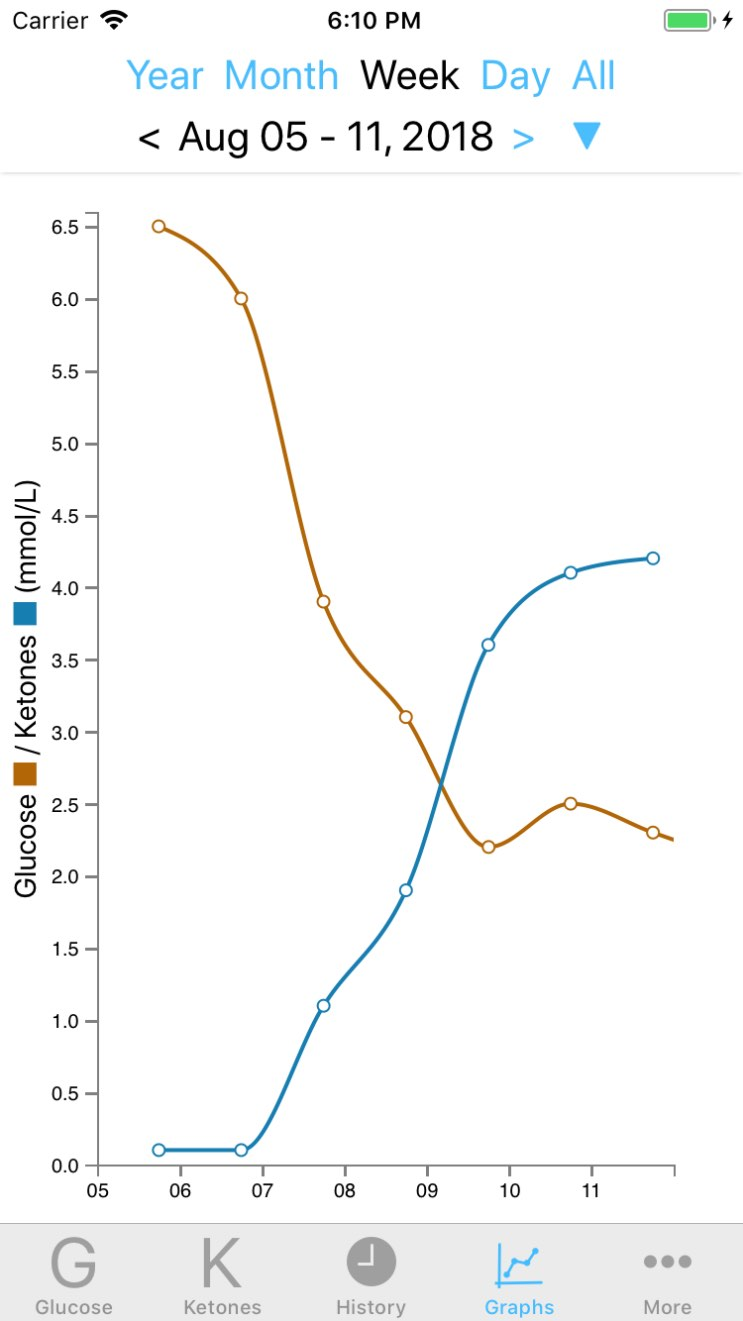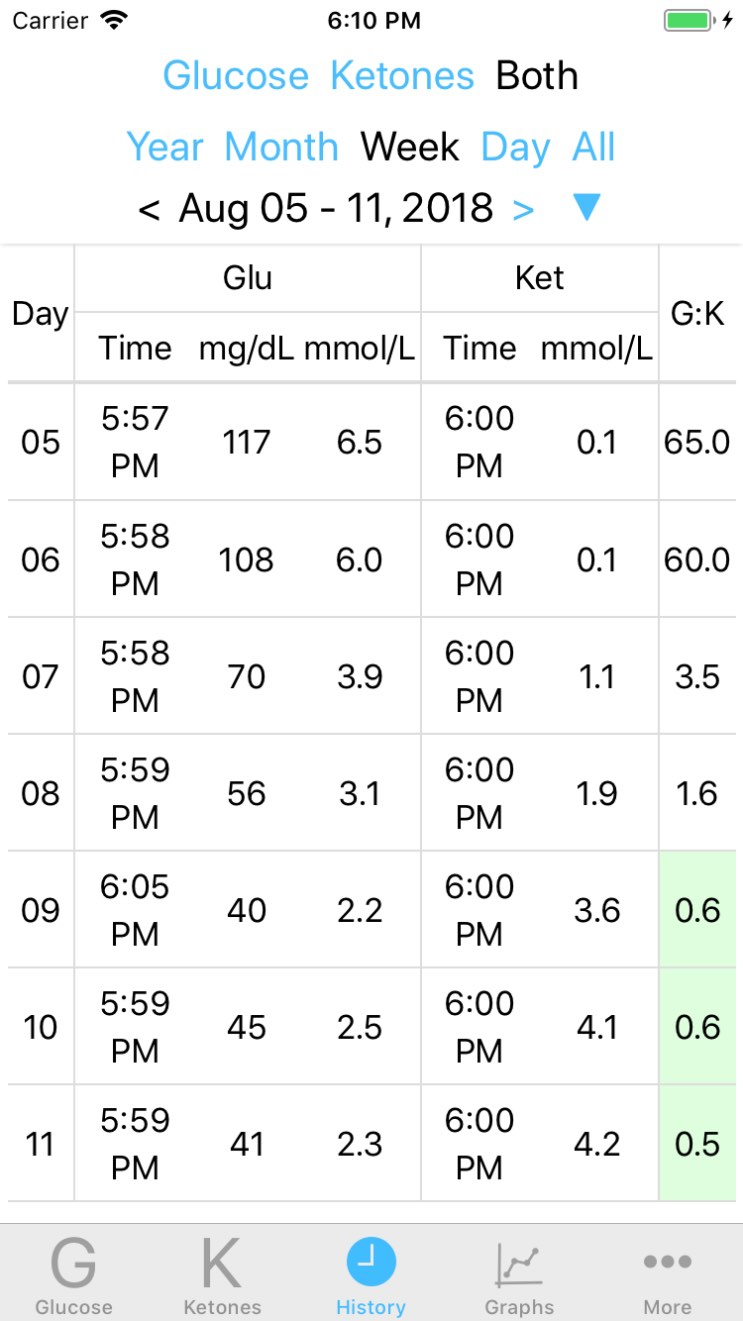Every once in a while I get a question asking: What is the GKI? What is g:k? Why is it green sometimes in the "Both" table.

This is the authoritative article on GKI:

There's a lot of math in it but a lot of it deals with how to convert glucose values from mg/dL to mmol/L. (They use mM but as far as I can tell that is equivalent to mmol/L.) The GKI or g:k is simply glucose in mmol/L divided by ketones in mmol/L.

The image below is similar to the one displayed in the article except made from a Ketologger screen capture.This is a glucose vs. ketone graph.

Glucose starts high and drops to a low level. Ketones start low and rise to a level someone might achieve when in nutritional ketosis.

Although I'm working on adding GKI graphing to Ketologger one of the reasons that I didn't from the start is that by looking at the glucose vs. ketone graph you can see a visual representation of the GKI. I'll explain below.

On the left of the graph in the screenshot above you see high/normal glucose and low ketones. This represents someone who is eating carbs and not in ketosis. Since glucose is high and ketones low you'll get a number higher than one for the GKI. (Eg. 6 divided by 1 gives you 6. Definitely larger than 1.)

As the graph moves to the right it represents someone who has lowered their carb intake enough so that ketones start to rise.

Near the center is where glucose and ketones meet. This is where the GKI is 1.0. When glucose in mmol/L equals ketones in mmol/L. Any number divided by itself is one. (Eg. 2.5 divided by 2.5 = 1.0)

Going rightward ketones have surpassed glucose. Most likely this person is in strict low carb or even maybe doing extended fasting. (Glucose has dipped quite a bit below what is considered normal fasting glucose for a non diabetic. For someone fasting or maybe even extermely low carb this may feel ok but it would probably be unpleasant to say the least for someone who is not fat adapted.) In this case glucose is a smaller number than ketones so when you divide glucose by ketones you get a number that is less than 1. That is a fraction. For eg. 2 divided by 4. That gives you on half which is 0.5.

To see that the GKI is less than one you can simply take look at the graph and see that ketones are above glucose. Or you can do the division of glucose by ketones and have the mathematical "picture" and know when the GKI is below 1.0.This is the "Both" history view in Ketologger which pairs up glucose and ketone readings. This matches the graph in the screenshot above. The GKI/G:K is displayed on the right. A G:K of less than or equal to 1.0 is highlighted in green.

In the "Both" table Ketologger pairs up the closest readings of glucose and ketones and calculates the GKI (by dividing glucose by ketones in mmol/L). You'll see it in green when it's at or below 1.0.

As mentioned I'm working on adding the GKI graph on it's own which will appear in a future release.